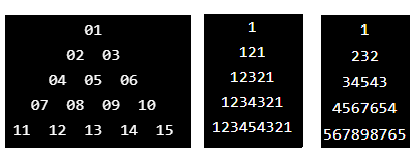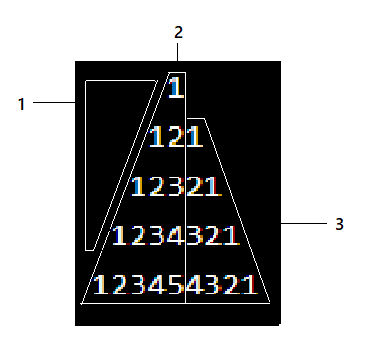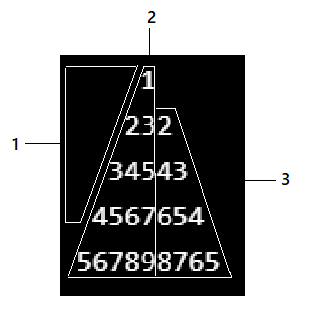New update is available. Click here to update.

# The Number Triangle

## Introduction

Writing programs to print different patterns using programming languages is an excellent way to improve coding skills. Numeric patterns are pretty interesting and are very easy to code. The Number triangle is one such numeric pattern that consists of numbers arranged in a way that represents a triangle. In this blog, let us learn how to print the following three number triangles.## Approach

All numbers in a number triangle have a relation with the number preceding it or following it. We use a for loop to traverse through each row. The given pattern is split into two or more patterns if possible. Each such pattern will need a for loop of its own.

### Pattern1

``````#include<stdio.h>
int main()
{
int n, i, j, k, a=1;
printf("Enter the number of rows\n");
scanf("%d", &n);
for(i=1; i<=n; i++)
{
for(k=n-1; k>=i; k--)
printf("  ");

for(j=0; j<i; j++)
printf(" %02d ", a++);

printf("\n");
}
}``````

Output:

``````Enter the number of rows
5
01
02  03
04  05  06
07  08  09  10
11  12  13  14  15 ``````

1. The number of rows to be printed is taken as input from the user. A for loop runs n times to keep track of the row it prints.

2. The first for loop is to print the spaces before every starting number in each row.

3. The second for loop is to print the number itself. Variable a holds this value and is incremented each time it is printed.

### Pattern2

``````#include<stdio.h>
int main()
{
int n,i,j,m;
printf("Enter the number of rows\n");
scanf("%d",&n);
for(i=1;i<=n;i++)
{
for(int k=n-1;k>=i;k--)
printf(" ");

for(j=1;j<=i;j++)
printf("%d",j);

for(m=i-1;m>0;m--)
printf("%d",m);

printf("\n");
}
}``````

Output:

``````Enter the number of rows
5
1
121
12321
1234321
123454321``````

The main for loop runs to print each row. The given pattern is split as follows:1. The first inner for loop is to print the spaces before every starting number in each row (section 1).

2. The second inner for loop is to print section 2.

3. The third inner for loop is to print section 3.

### Pattern3

``````#include<stdio.h>
int main()
{
int n,i,j,m,a;
printf("Enter the number of rows\n");
scanf("%d",&n);
for(i=1;i<=n;i++)
{
for(int k=n-1;k>=i;k--)
printf(" ");
a=i;
for(j=0;j<i;j++)
printf("%d",a++);
a--;
for(m=i-1;m>0;m--)
printf("%d",--a);
printf("\n");
}
}``````

Output:

``````Enter the number of rows
5
1
232
34543
4567654
567898765``````

The main for loop runs to print each row. The given pattern is split as follows:1. The first inner for loop is to print the spaces before every starting number in each row (section 1).

2. The second inner for loop is to print section 2.

3. The third inner for loop is to print section 3.

You can practice by yourself on Online C editor for good understanding.

## Complexity Analysis

• Time Complexity: O(n^2) is the time complexity. This is because the main loop has nested loops. This means that every statement in the nested loop will run n x m times, where m<=n.

• Space Complexity: No new data structure was created to print the patterns. So, the space complexity is constant.

## FAQs

1. What is the purpose of using loops to print number triangles?

Multiple for loops are used to print patterns. The first outer loop is used to iterate through the number of rows, and the inner loop is used to print the columns.

2. Name some types of Number triangles.

Pascal's triangle is a triangular array of binomial coefficients. Floyd's triangle is a right-angled triangle that contains consecutive natural numbers. The Fibonacci triangle or Hosoya’s triangle is a triangular arrangement of numbers based on Fibonacci numbers.

## Key Takeaways

This article extensively discusses how to construct number triangles in C. We hope that this blog has helped you enhance your knowledge about printing number triangles in C. If you would like to learn more, check out our articles on the Coding Ninjas Library. Do upvote our blog to help other ninjas grow. Happy Coding!